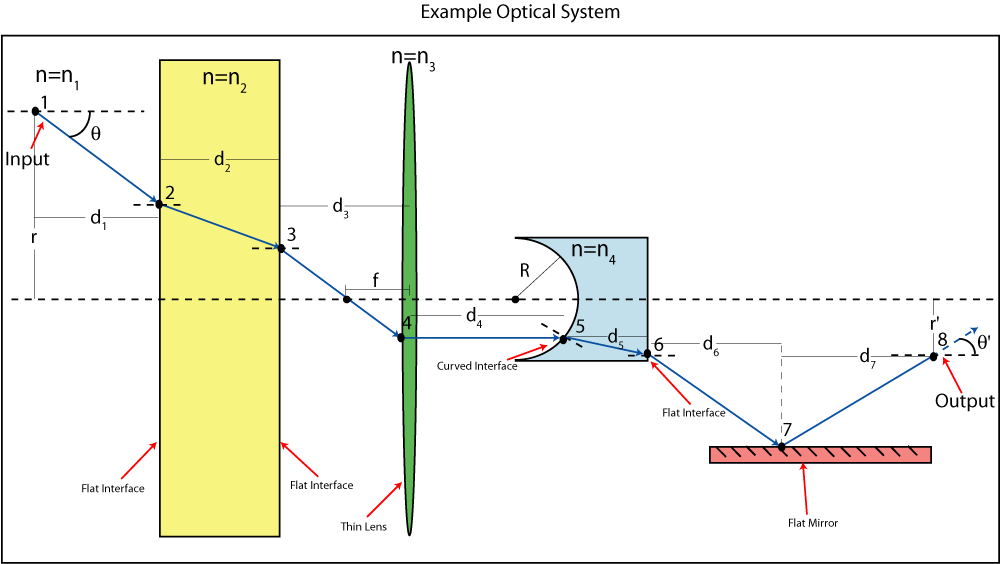BRIGHAM YOUNG UNIVERSITY
 Search BYUContact   |   Help[expand all...]
[COLLAPSE ALL...]# ABCD Matrices Tutorial

## Definition: a 2-by-2 matrix associated with an optical element which characterizes the element's effect on a light ray

#### The input beam is described by a vector (with some transverse offset r and offset θ angle from a reference axis) An ABCD matrix which accurately describes the optical element is formed (see below for more information on how to form the ABCD matrices) The ABCD matrix is multiplied by the input vector The result is a vector which describes the output beam# ABCD Matrices for simple optical components

#### In short an ABCD matrix allows the output of an optical element to be written in terms of its input. The hard part about ABCD matrix analysis is coming up with the appropriate ABCD matrices for the elements in the system to be analyzed. Below is the derivation of the ABCD transfer matrix of a beam traveling through free space or in a medium of constant refractive index. The derivations of the transfer matrices for other simple optical elements follow the same pattern and are relatively straightforward. The detailed derivations of the transfer matrices of other simple optical elements will not be discussed in this tutorial, but a table is included which gives the general form of the transfer matrices for several optical elements.# Table of ABCD Matrices for simple optical components

Element Matrix Remarks
Propagation in free space or in a medium of constant refractive indexd = distance
Refaction at a flat interfacen1 = initial refractive index
n2 = final refractive index
Refaction at a curved interfaceR = radius of curvature, R > for convex (centre of curvature after interface)
n1 = initial refractive index
n2 = final refractive index
Reflection from a flat mirrorIdentity matrix
Reflection from a curved mirrorR = radius of curvature, R > 0 for convex
Thin lensf = focal length of lens where f > 0 for convex/positive (converging) lens. Valid if and only if the focal length is much greater than the thickness of the lens

# ABCD Matrix Example

#### Below is an example of a number of simple optical elements combined in order to form an optical system. Following the diagram there is a short discussion on how to obtain an ABCD matrix which characterizes the entire system. Or if you would like to use an ABCD calculator for your own system click here## Note: Each element in the numbered list corresponds with a 2 by 2 transfer matrix in the expression below (they are numbered respectively)

#### ☆ ☆ ☆ One of the properties of ABCD matrices that makes them extremely useful is that a system of several optical elements can be described by a single ABCD matrix. This ABCD matrix is the product of the ABCD matrices of each of the optical elements in the system. ☆ ☆ ☆

1. Transfer matrix of the medium between points 1 and 2 (with constant index of refraction n1)
2. Transfer matrix of the flat interface at point 2
3. Transfer matrix of the medium between points 2 and 3 (with constant index of refraction n2)
4. Transfer matrix of the flat interface at point 3
5. Transfer matrix of the medium between points 3 and 4 (with constant index of refraction n1)
6. Transfer matrix of the thin lens with focal length f and index of refraction n3
7. Transfer matrix of the medium between points 4 and 5 (with constant index of refraction n1)
8. Transfer matrix of the curved interface at point 5
9. Transfer matrix of the medium between points 5 and 6 (with constant index of refraction n4)
10. Transfer matrix of the flat interface at point 6
11. Transfer matrix of the medium between points 6 and 7 (with constant index of refraction n1)
12. Transfer matrix of the flat mirror at point 7
13. Transfer matrix of the medium between points 7 and 8 (with constant index of refraction n1)Maintained by ECEn IMMERSE Web Team.